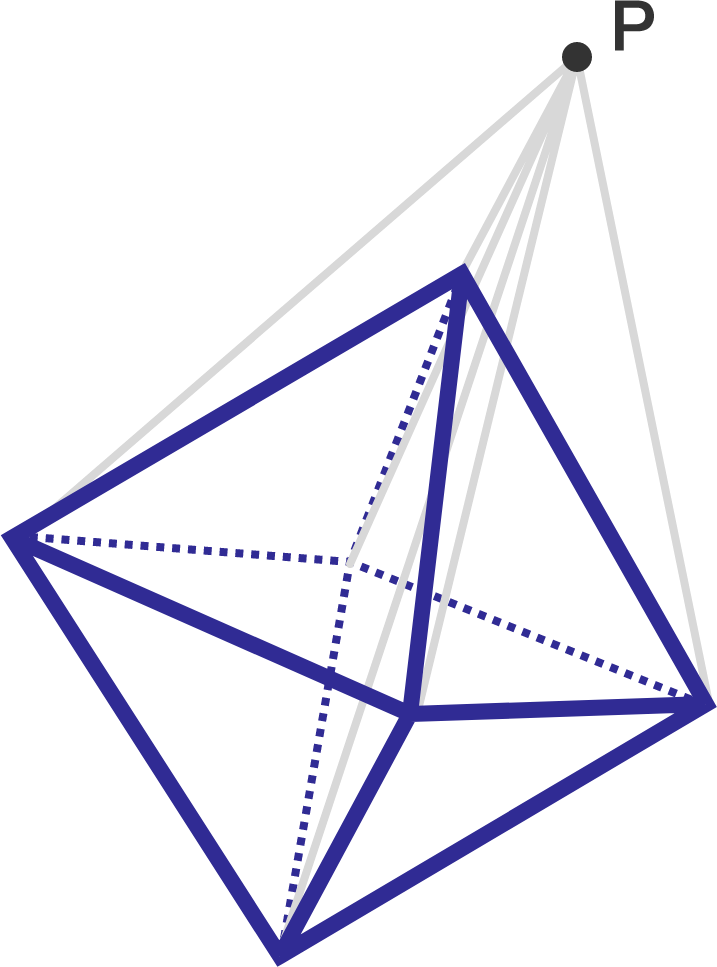# Lost in space

Geometry Level 3

A point $P$ in $\mathbb{R}^{3}$ has distances of $3,7,8,9$ and $11$ from five of the vertices of a regular octahedron. If the distance from the sixth vertex of the octahedron to $P$ is $\sqrt{a}$, where $a$ is a positive, square-free integer, then find $a$.×

Problem Loading...

Note Loading...

Set Loading...